Learn all Concepts of Chapter 5 Class 10 (with VIDEOS). Check - Arithmetic Progressions - Class 10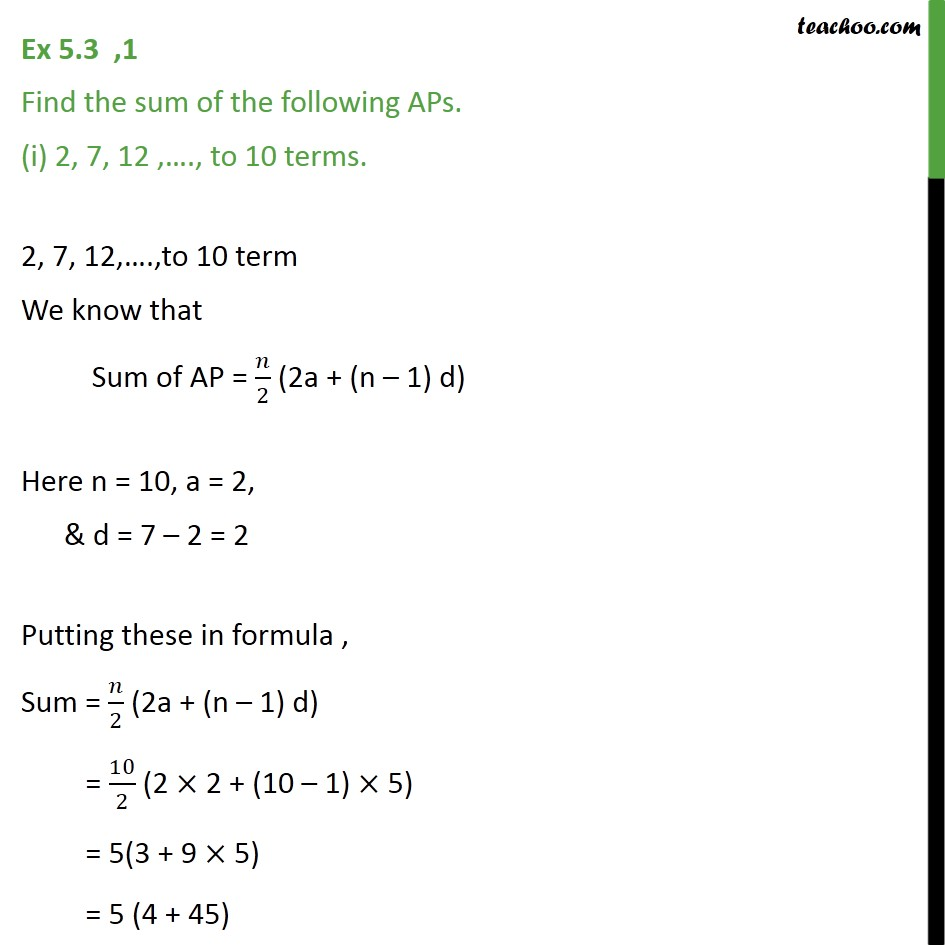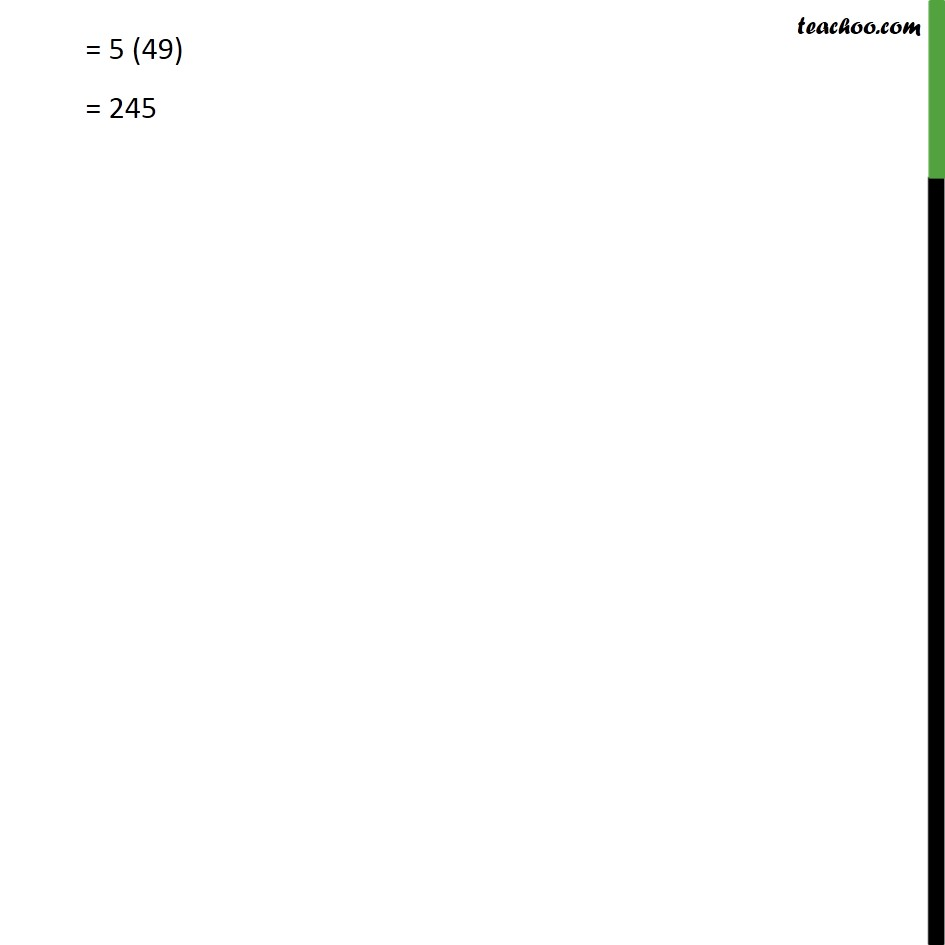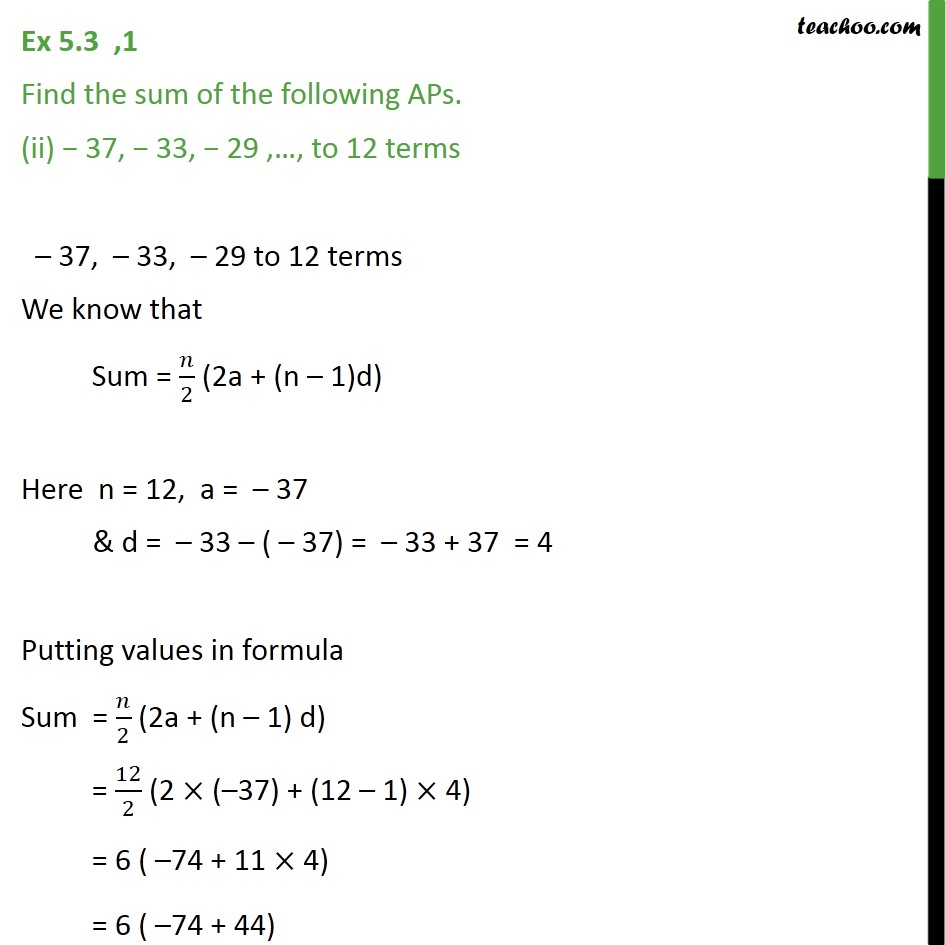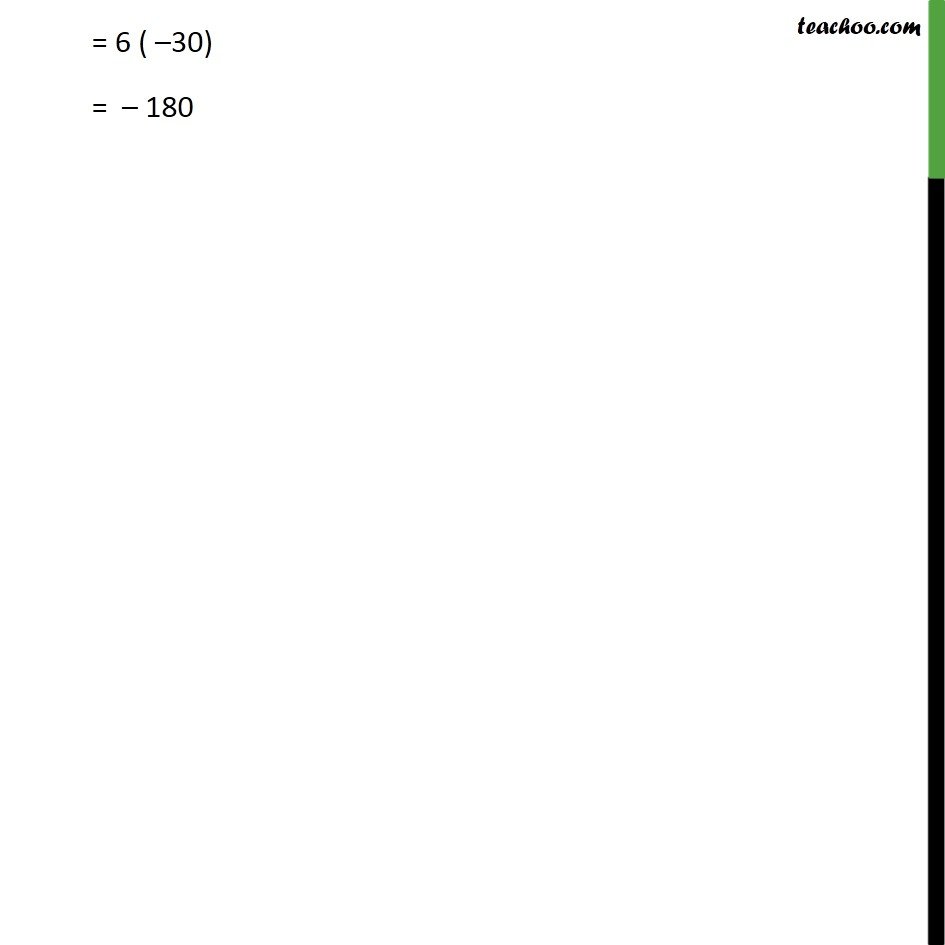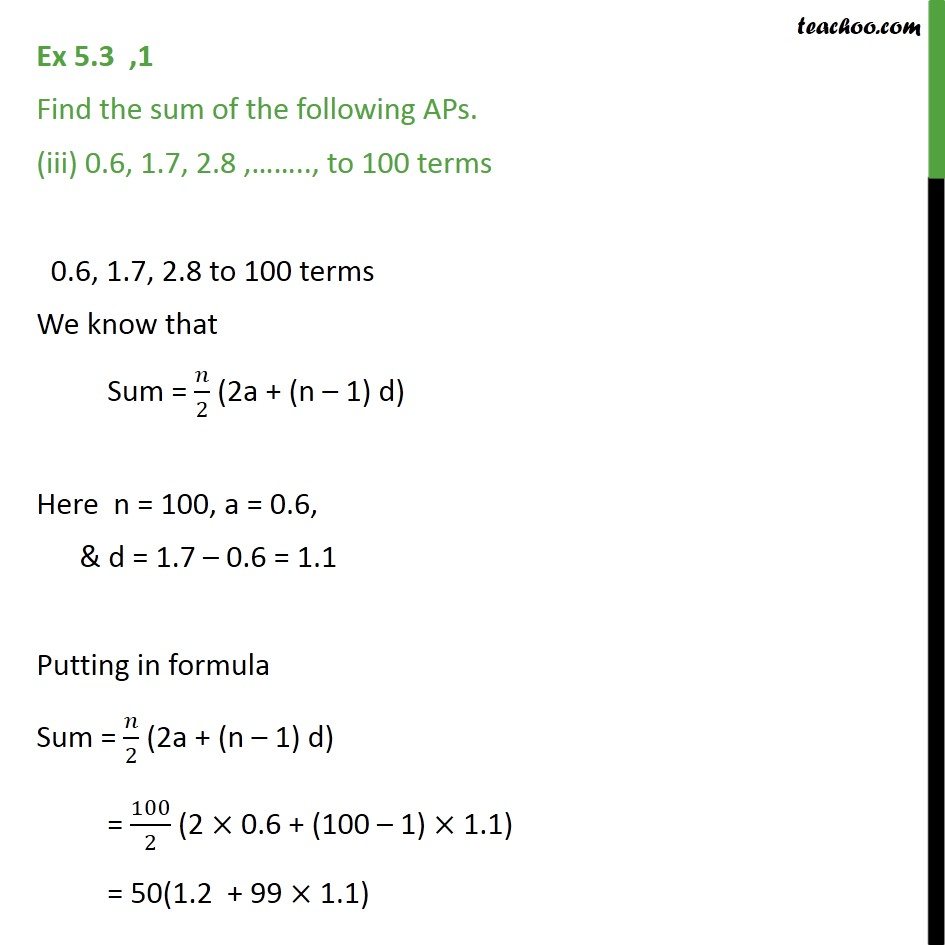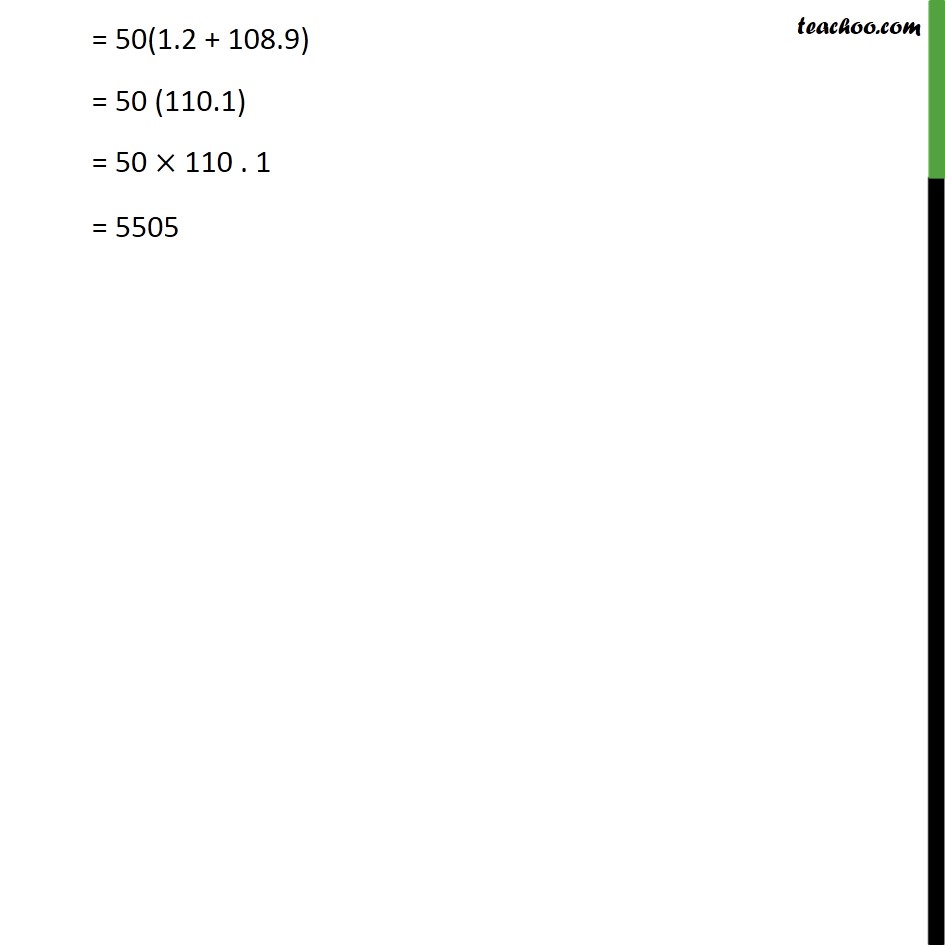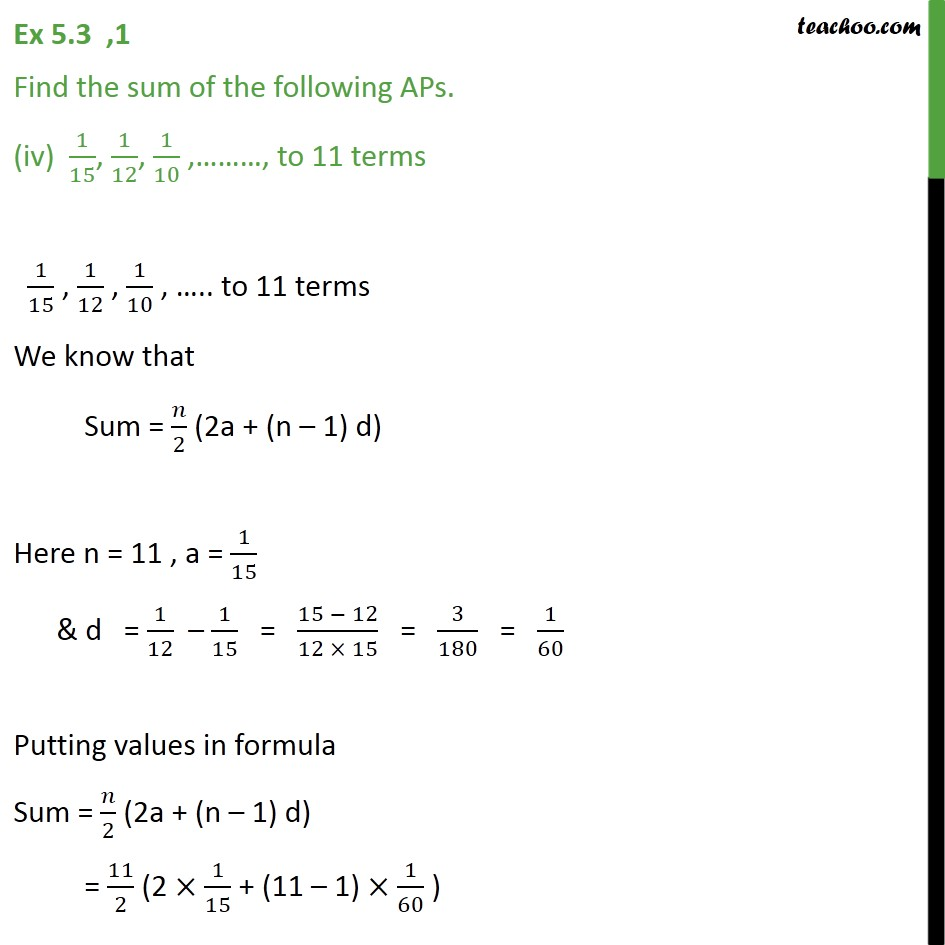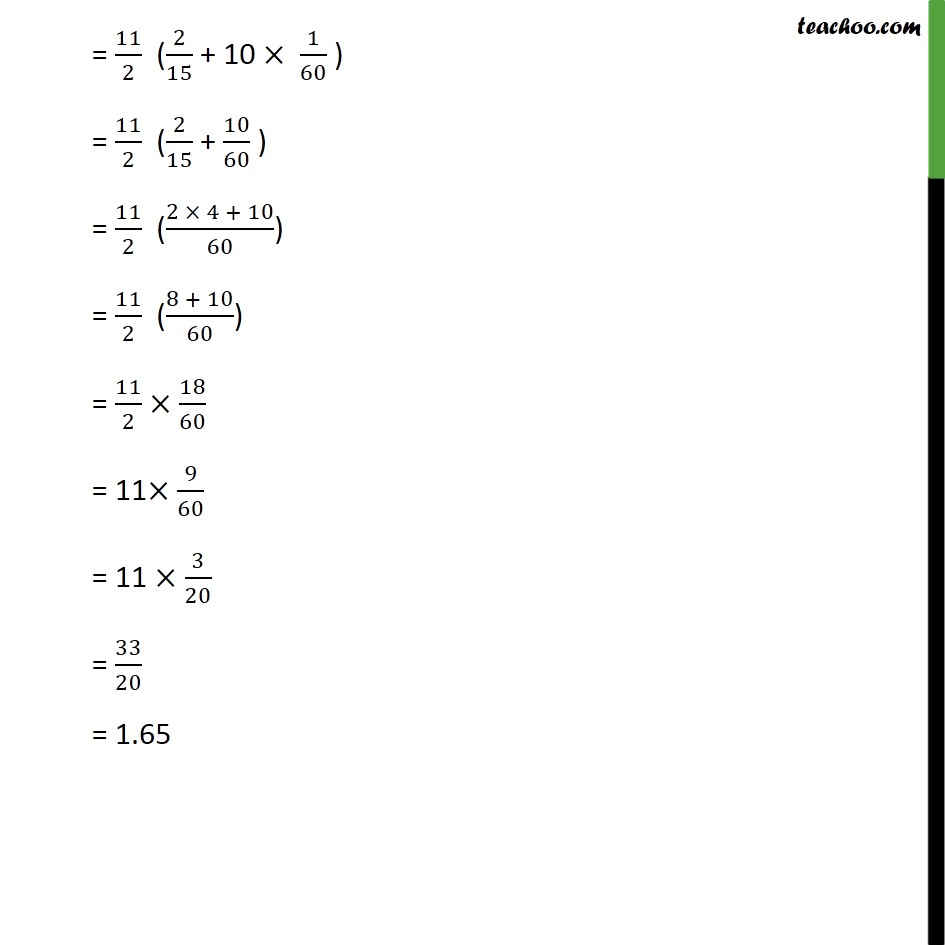1. Chapter 5 Class 10 Arithmetic Progressions
2. Serial order wise
3. Ex 5.3

Transcript

Ex 5.3 ,1 Find the sum of the following APs. (i) 2, 7, 12 , ., to 10 terms. 2, 7, 12, .,to 10 term We know that Sum of AP = /2 (2a + (n 1) d) Here n = 10, a = 2, & d = 7 2 = 2 Putting these in formula , Sum = /2 (2a + (n 1) d) = 10/2 (2 2 + (10 1) 5) = 5(3 + 9 5) = 5 (4 + 45) = 5 (49) = 245 Ex 5.3 ,1 Find the sum of the following APs. (ii) 37, 33, 29 , , to 12 terms 37, 33, 29 to 12 terms We know that Sum = /2 (2a + (n 1)d) Here n = 12, a = 37 & d = 33 ( 37) = 33 + 37 = 4 Putting values in formula Sum = /2 (2a + (n 1) d) = 12/2 (2 ( 37) + (12 1) 4) = 6 ( 74 + 11 4) = 6 ( 74 + 44) = 6 ( 30) = 180 Ex 5.3 ,1 Find the sum of the following APs. (iii) 0.6, 1.7, 2.8 , .., to 100 terms 0.6, 1.7, 2.8 to 100 terms We know that Sum = /2 (2a + (n 1) d) Here n = 100, a = 0.6, & d = 1.7 0.6 = 1.1 Putting in formula Sum = /2 (2a + (n 1) d) = 100/2 (2 0.6 + (100 1) 1.1) = 50(1.2 + 99 1.1) = 50(1.2 + 108.9) = 50 (110.1) = 50 110 . 1 = 5505 Ex 5.3 ,1 Find the sum of the following APs. (iv) 1/15, 1/12, 1/10 , , to 11 terms 1/15 , 1/12 , 1/10 , .. to 11 terms We know that Sum = /2 (2a + (n 1) d) Here n = 11 , a = 1/15 & d = 1/12 1/15 = (15 12)/(12 15) = 3/180 = 1/60 Putting values in formula Sum = /2 (2a + (n 1) d) = 11/2 (2 1/15 + (11 1) 1/60 ) = 11/2 (2/15 + 10 1/60 ) = 11/2 (2/15 + 10/60 ) = 11/2 ((2 4 + 10)/60) = 11/2 ((8 + 10)/60) = 11/2 18/60 = 11 9/60 = 11 3/20 = 33/20 = 1.65

Ex 5.3